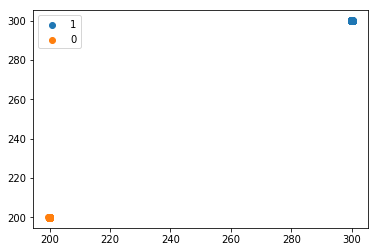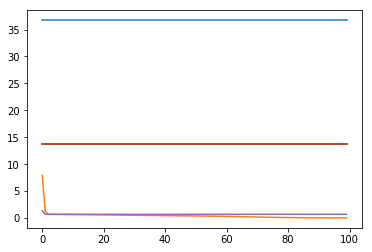# Using network to do classification on mixture of gaussian, weird behaviour

Hey; I construct a very simple classification model to classify mixture of gaussian.

In this case, bivariate Gaussian. The data close to mode one has label 0 and data close to mode two has label 1.

Here is how I generate train samples

``````from torch.distributions.multivariate_normal import MultivariateNormal
m1 = MultivariateNormal(torch.zeros(2) + 300,torch.eye(2) * .01)
m2 = MultivariateNormal(torch.zeros(2) + 200.,torch.eye(2) * .01)

x1 = m1.sample((1000,)) # mode 1
x2 = m2.sample((1000,)) # mode 2

c1 = torch.zeros(1000) # labels for mode 1
c2 = torch.ones(1000) # labels for mode 2

x = torch.cat([x1,x2],dim=0)
c = torch.cat([c1,c2],dim=0).view(-1,1)
``````

The train sample look like thisNow I construct a simple classifier

``````class Classifier(nn.Module):
def __init__(self,num_in_dim=2,num_hidden=100):
super(Classifier, self).__init__()

self.fc1 = nn.Sequential(
nn.Linear(num_in_dim, num_hidden),
nn.ReLU(inplace=True),
nn.Linear(num_hidden, 1),
nn.Sigmoid())

def forward(self,x):
return self.fc1(x)
``````

Now I set up my training as

``````net = Classifier()
criterion = nn.BCELoss()
for i in range(100):
a = net(x)
loss = criterion(a, c)
loss.backward()
optimizer.step()
if i % 100 == 0:
print(loss.item())
``````

The weird behaviour I observe is that. Here are the train loss. I use the same set of batch samples train the same network with only initial parameters different. Each line is a one initialization of network. We see that sometimes the network doesn’t learn at all but some times it works really well. Though, there are many theory on optimization about local minimal etc. But I think example like this is too trivia and same thing happens even I use linear neural network.I tried a few approaches using different weight init methods etc., but the main issue seems to be the loss in numerical precision using `sigmoid` + `nn.BCELoss`.
If you remove the sigmoid in your model and use `nn.BCEWithLogitsLoss` as your criterion (which uses the LogSumExp trick for stability), your model seems to converge in all runs.

Thanks for the reply; so I guess that this is also true that using `nn.CrossEntropyLoss` is more numerically stable than using `nn.LogSoftmax` + `nn.NLLLoss` ?

No, internally `F.nll_loss(F.log_softmax)` will be used as seen in this line of code.
However, `F.log_softmax` is more numerically stable than `F.log(F.softmax)`.

I see. Thanks. So in the neural network with binary classification, `nn.BCEWithLogitsLoss` as my loss function and in my neural network has no activation in the last layers. In the test time, I just manually apply sigmoid function to my output from neural network

Yes, you could do that to e.g. apply a probability threshold to get the predicted class.